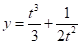Chapter 10, Problem 41RE

Chapter
Section
Textbook Problem

# Find the area of the surface obtained by rotating the given curve about the x-axis.41.,, 1 ≤ t ≤ 4

To determine

To find: The area of the surface obtaining by rotating the curve x=4t and y=t33+12t2 for 1t4 about x-axis.

Explanation

Given:

The parametric equation for the variable x is as follows.

x=4t (1)

The parametric equation for the variable y is as follows.

y=t33+12t2 (2)

Calculation:

Differentiate the equation (1) with respect to t .

dxdt=4×12(t12)

dxdt=2(t12)

dxdt=2t (3)

Differentiate the equation (2) with respect to t .

dydt=3t2t3

dydt=t21t3 (4)

Surface area is determined for the limits of 1 to 4 .

Calculate the surface area the curve rotating about x axis using the formula.

S=142πy(dxdt)2+(dydt)2dt (5)

Substitute the expressions from equation (2), (3) and (4) into equation (5).

S=142πy(dxdt)2+(dydt)2dt=142π(t33+12t2)(2t)2+(t21t3)2dt=142π(t33+12t2)4t+t4+1t6(2t2×1t3)dt

S=142π(t33+12t2)4t+t

### Still sussing out bartleby?

Check out a sample textbook solution.

See a sample solution

#### The Solution to Your Study Problems

Bartleby provides explanations to thousands of textbook problems written by our experts, many with advanced degrees!

Get Started

#### Find the limit. limxln(sinx)

Single Variable Calculus: Early Transcendentals, Volume I

#### π does not exist

Study Guide for Stewart's Multivariable Calculus, 8th

#### Draw a scatterplot for which r = 1.

Introduction To Statistics And Data Analysis